# Mass Spectrometry Basics for the Absolute Novice

## What is a mass spectrometer?

Mass spectrometers weigh atoms and molecules.1

• Mass spectrometers weigh atoms and molecules.1
• A mass spectrometer produces charged particles (ions) from the chemical substances that are to be analyzed.
• The electric charge is like a “handle” that allows the electric and magnetic fields to move the charged particles around so that we can measure the mass (“weight”)1 of the charged particles.

Atoms that make up chemical compounds have characteristic masses. Mass spectrometers use electric and magnetic fields to measure the masses of atoms and molecules.

## What are mass spectrometers used for?

Mass spectrometers are used to determine what chemicals are present in a sample (“qualitative analysis”), and also how much of each chemical is contained in a sample (“quantitative analysis”). Mass spectrometers have many applications in a wide range of fields including forensics, environmental analysis, biology, quality control and troubleshooting, space exploration, and art conservation.

## How do mass spectrometers work?

All mass spectrometers have at least three components: (1) an ion source, (2) a mass analyzer, and (3) a detector. The ion source makes charged particles that are separated by the mass analyzer, and the detector records the information that is sent to the computer to be interpreted.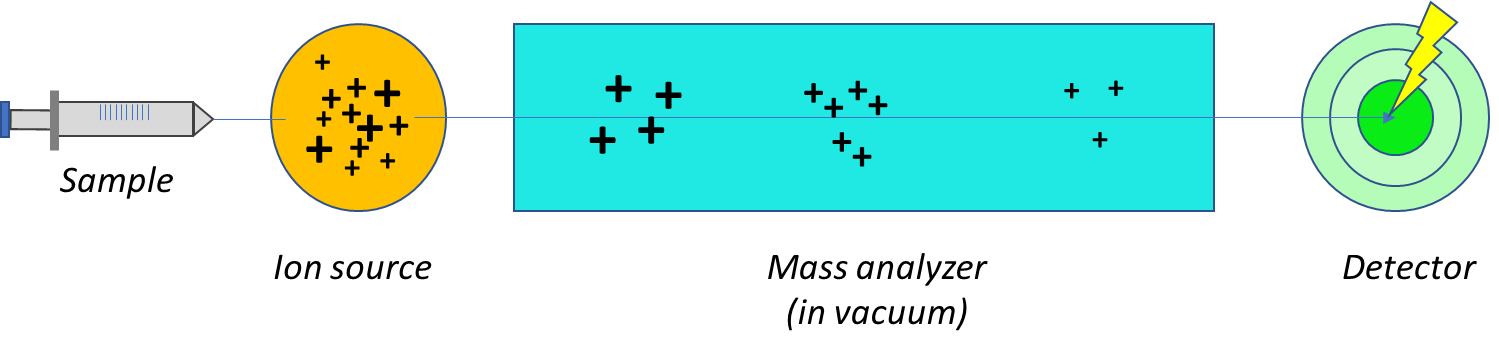The three components found in all mass spectrometers.

### Ion Sources

The ion source creates electrically charged particles (“ions”) from the atoms and molecules in the sample. There are many different kinds of ion sources – some operate in a high vacuum, and some create ions at atmospheric pressure. The different sources will be described in more detail in a separate article. Ions can be made from gases, liquids or solids, but the ions are always introduced into the mass analyzer in a gaseous state.

##### How do we make ions?

There are many ways to make ions. In a vacuum, some common ion sources are based on a:

• High-energy electron beam (Electron ionization: EI)
• High-energy electron beam in gas with chemical reactions (Chemical ionization: CI)
• Ultraviolet light (Photoionization: PI)
• Laser beam striking the sample (Matrix-assisted laser desorption: MALDI)

You can also make ions at atmospheric pressure and transfer them into a vacuum to be separated in the mass analyzer. For the purposes of this basic discussion, we will only consider electron ionization (EI).

### The mass analyzer

Sample ions are always introduced into the mass analyzer in a vacuum2. If the sample was not in a vacuum, the charged particles would bump into gas molecules, and we could not move them effectively with the electric and magnetic fields. The mass analyzer actually separates ions by their mass-to-charge ratio, symbolized as “m/z“ (in italics), but for the purposes of this discussion, let’s assume that all of the ions have a single charge. There are many different kinds of mass analyzers. The most common types are quadrupoles, magnetic sectors, time-of-flight mass spectrometers, and trapped-ion mass spectrometers.

##### Example: a time-of-flight (TOF) mass analyzer

The easiest mass analyzer to understand is the time-of-flight (TOF) A time-of-flight mass spectrometer uses electric fields to separate ions with different masses. You can think of it as a race track where the smaller particles move faster than the larger particles – like a sprinter racing a sumo wrestler. Both might have equal energy, but the smaller person will move faster than the larger person.

Here’s another way to think about it: you can throw a baseball faster than you can throw a bowling ball.

In the TOF analyzer, a short electrical pulse is used to start the ions traveling down a tube. The smallest ions (the ones with the lowest mass) move faster than the larger ions, so they arrive at the detector first. The time it takes for an ion to travel down the “flight tube” is related to the mass of the ion.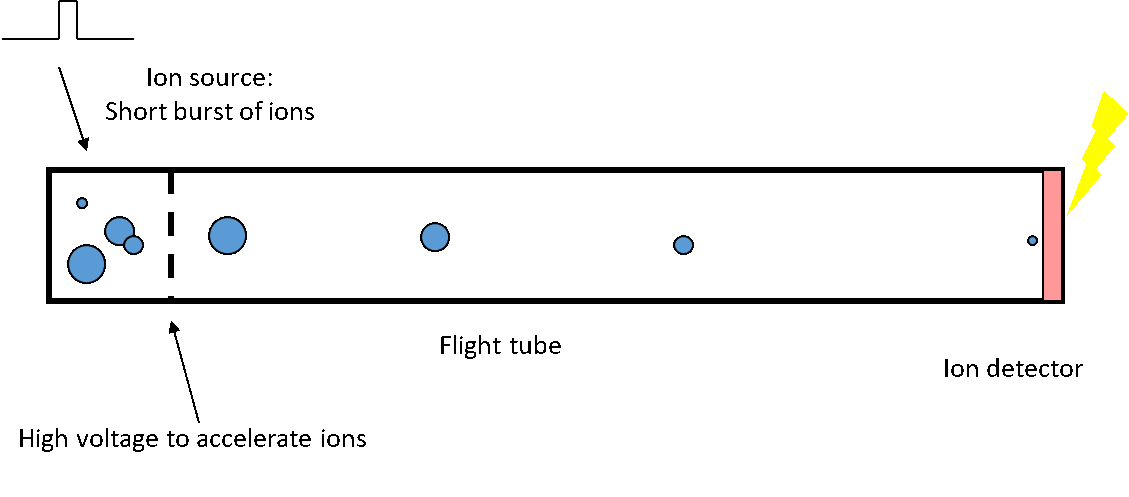A simplified diagram of a time-of-flight mass spectrometer.
In simplified physics terms

If all of the ions have the same charge and are accelerated with the same electrical potential V, they will all have the same kinetic energy Ek.

E k = q V, but kinetic energy is 1/ 2 mv 2.

So, if all of the ions have the same kinetic energy (qV is constant), ions with a larger mass must move slower (v is small), and ions with a smaller mass must move faster (v is large). The flight time for a given mass is inversely proportional to the square root of the mass/charge ratio.

The physics for any mass analyzer can be derived from the Lorentz force:

F = ma = q(E + v × B)

Where F is force, m is mass, a is acceleration, q is the electric charge, E is the electric field, and B is the magnetic field.

## The detector

The detector records the signals from the ions and sends that information to the computer. In a time-of-flight mass spectrometer, the detector creates an electrical signal every time an ion strikes the detector. The information about the time the ion strikes the detector and the number of ions that strike the detector at each time interval is used by the computer to create the mass spectrum.

### What does a mass spectrum look like?

The mass spectrum is a graph that displays the mass-to-charge ratio (m/z) on the x-axis and the relative intensity (number of ions detected) on the y-axis. An electron ionization (EI) mass spectrum of water is shown below. We’ll explain what this tells us in a minute.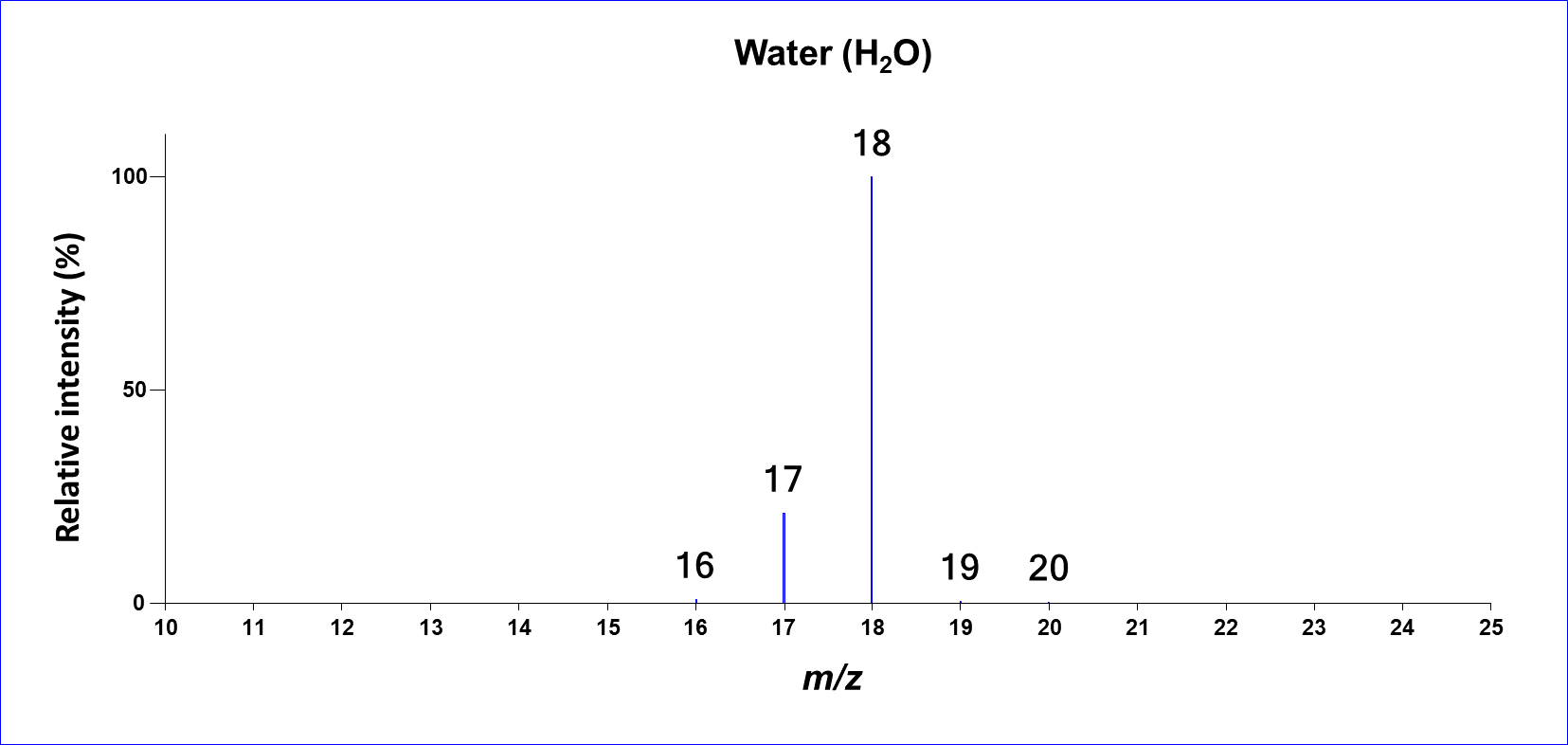An electron ionization mass spectrum of water.

### Mass

Atoms have characteristic masses that represent the amount of matter in an atom of each element. For example, the most common forms of carbon, hydrogen, and oxygen on earth have integer masses of 12, 1, and 16, respectively, but the masses aren’t exactly integers3.

Carbon (12C) has a mass of 12 (12.0000)

Hydrogen (1H) has a mass of 1 (1.0078)

Oxygen (16O) has a mass of 16 (15.9949)

Nitrogen (14N) has a mass of 14 (14.0031)

Water (H2O) has two hydrogen atoms and one oxygen atom, so the integer mass of water is

Mass of water = (2 x 1) + (16 x 1) = 18

We can see that the largest peak in the water mass spectrum is at m/z 18. What are the other peaks in the mass spectrum?

### Isotopes

Atoms are made up of protons (positive charge), neutrons (uncharged) and electrons (negative charge). Here’s a schematic diagram of a helium (He) atom: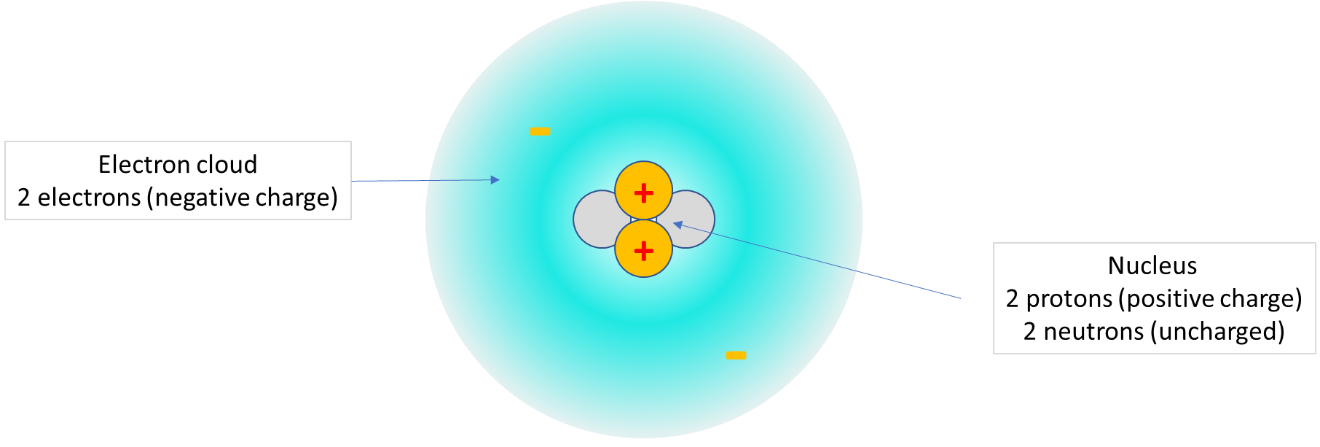A schematic diagram of a helium (He) atom.

Helium Atom Diagram

• 2 protons (+): mass = 1.007825
• 2 electrons (-): mass = 0.0005
• 2 neutrons (0): mass = 1.00866
• Exact mass = 4.002600
• Charge: 2 protons (+) + 2 electrons (-) = 0 charge

The mass of the helium atom is approximately 4, but it isn’t exactly an integer. The exact mass of the helium atom is 4.002600. The exact mass of the atom is smaller than the sum of the masses of the protons, neutrons and electrons because of the nuclear binding energy4. This is called the “mass defect”.

Not all atoms of a given element have the same number of neutrons, so some atoms of a particular element can have different masses. Atoms of an element that have different numbers of neutrons are called isotopes. Helium has another stable isotope, helium-3 (3He), that has one proton and 2 neutrons and an exact mass of 3.016029.  Helium-3 is very rare on earth, with a relative abundance of only about 0.000137%.

Most carbon on earth has 6 protons and 6 neutrons, so it has a mass of 12 (exactly). About 1.1% of the carbon on earth has 7 neutrons, and an integer mass of 6+7 = 13. Carbon-13 (13C) atoms have an exact mass of 13.003355.

The relative amounts of the different isotopes are additional information we can get from a mass spectrum. Some elements, such as chlorine and bromine have very distinctive isotope patterns that are easy to recognize in a mass spectrum: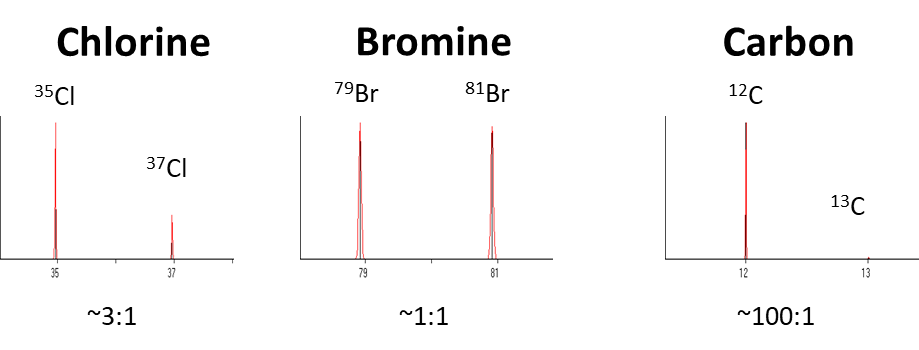Isotopes for chlorine, bromine, and carbon

A tiny fraction of the hydrogen on earth has an extra neutron, giving it an integer mass of 2 (let’s ignore the exact mass for now), and a fraction of the oxygen on earth has two extra neutrons, giving it a mass of 18. That’s why we see small peaks at m/z 19 and 20 in the mass spectrum of water. What are the other peaks at m/z 16 and 17?

### Fragment ions

As we explained above, an electron ionization source shoots a high-energy beam of electrons into a cloud of gas in a vacuum chamber. This knocks an electron off of the atom, creating an ion. If we knock an electron off of the helium atom in the diagram above, we have removed a negative charge. That means that we have two protons (+) and one electron (-), so there is a net positive charge. We show this with a plus sign, for example He+. The electron beam doesn’t have enough energy to break apart the nucleus of the atom, but it does have enough energy to break apart atoms in a molecule. Some of the water molecules were shattered when the electron beam hit them, so some of the ions are H2O+ (m/z 18), but some are OH+ (m/z 17) and some are O+ (m/z 16). Some are also H+ (m/z 1), but they aren’t shown in the water mass spectrum in the figure above.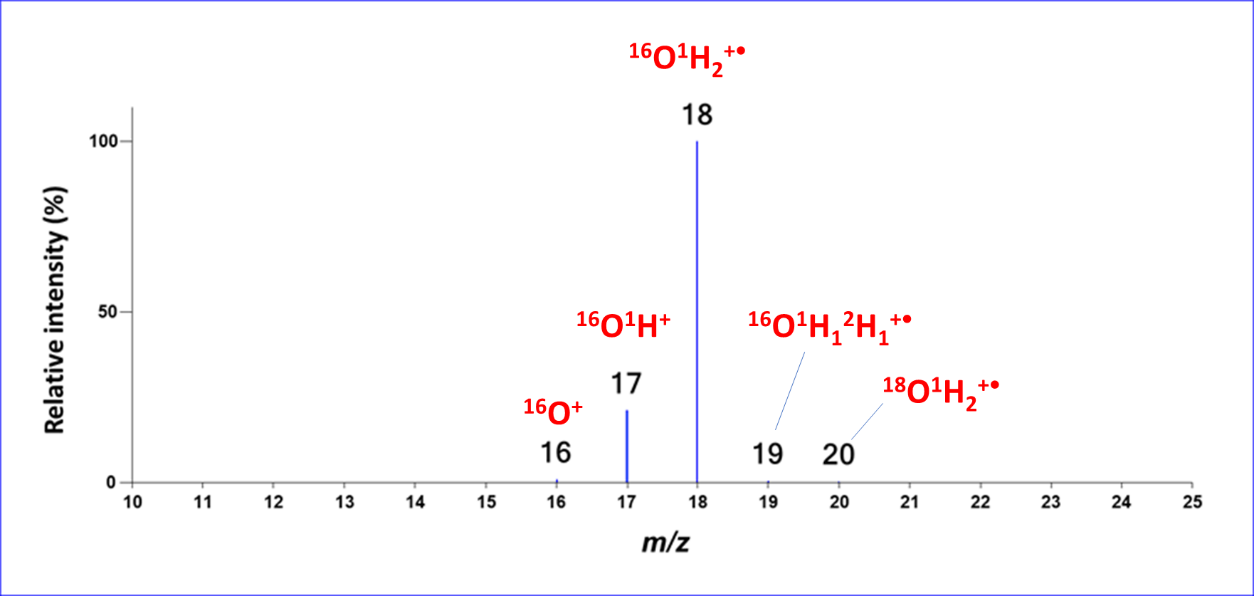Electron ionization mass spectrum of water with isotopes and fragments labeled.

Here’s an EI mass spectrum for a more complicated molecule: a drug of abuse.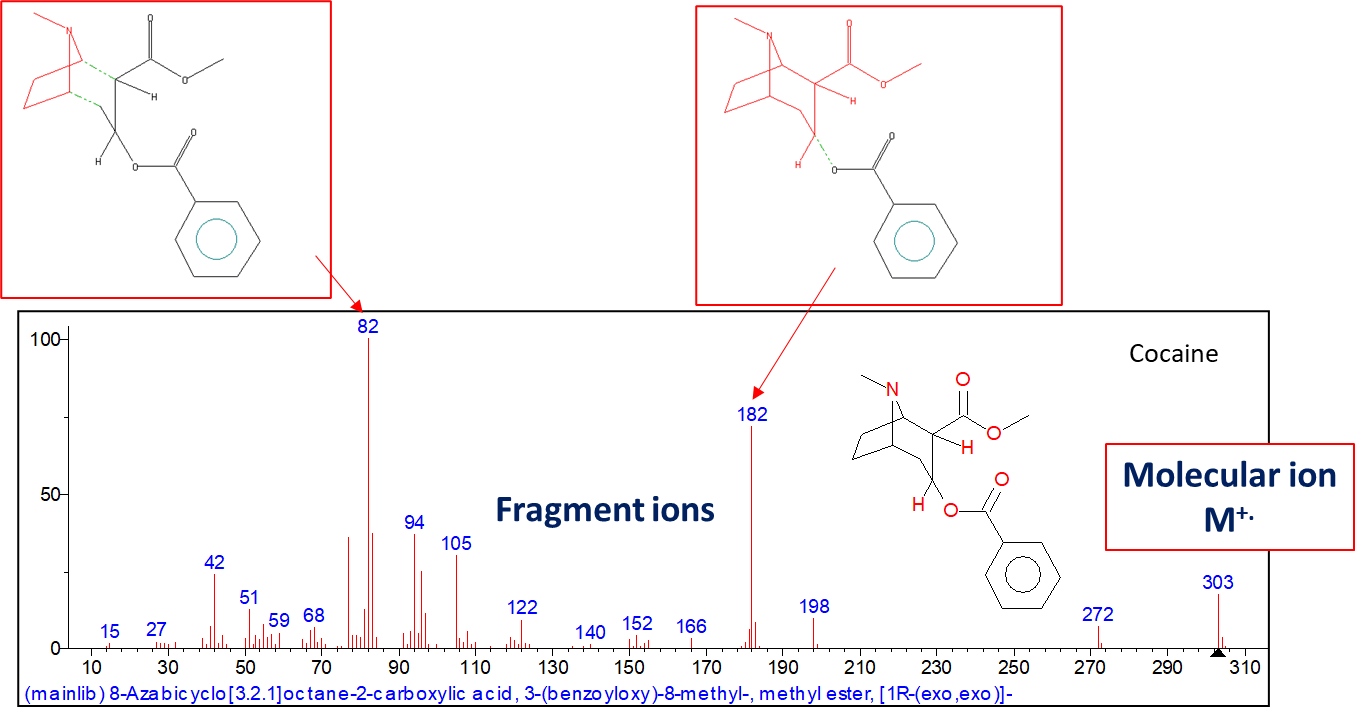An electron ionization mass spectrum of a drug of abuse.

The unfragmented molecule (C17H21NO4) is at m/z 303. This is called the molecular ion. The fragment ions represent different parts of the molecule. You can try to piece these “puzzle pieces” together to figure out the chemical structure. The most common way to identify molecules from EI mass spectra is to search databases of mass spectra to look for compounds with matching fragment patterns5. Databases are available with mass spectra for hundreds of thousands of chemical compounds.

### High resolution and exact masses

The most common mass spectrometers can only separate and detect ions with integer masses, as shown in the mass spectra above. These are called “low-resolution” mass spectrometers. “High-resolution” mass spectrometers such as the JEOL time-of-flight mass spectrometers can separate ions that have the same integer mass, but different exact masses. Having information about the exact mass can be very useful. Here’s an example:

Nitrogen (N2+) has an exact mass of 28.0061.Mass calculation for nitrogen

The exact mass of carbon monoxide (CO+) is 27.9949.Mass calculation for carbon dioxide

If you were given a gas with a mass of 28 and asked if you would be willing to inhale it, you might find yourself in the hospital if you made the wrong choice! However, if you were told that the gas has a mass of 28.0061, you’d be fine as long as you only inhaled it for a very short time.

Software programs can use the exact mass and isotope data from a mass spectrum to calculate the elemental composition of an unknown molecule. This can be very useful, especially if the compound you are trying to analyze does not have a mass spectrum in the databases.

Mass spectrometers don’t separate mixtures by themselves6. Mass spectrometers are often combined with equipment that separates compounds in mixtures before the compounds are introduced into the mass spectrometer. Gas chromatography (GC) and liquid chromatography (LC) are two of the most common separation methods that are combined with mass spectrometry in GC-MS and LC-MS systems. Compounds that are separated by a GC or LC are introduced into the mass spectrometer at different times. The mass spectrometer data system records a series of mass spectra as the different compounds are eluted from the chromatograph.

### Notes

 We really aren’t measuring weight of course, because gravity isn’t playing a role in the measurement.  We’re measuring mass (actually, the mass-to-charge ratio). Mass is independent of gravity.

 There is another kind of analyzer called an ion mobility spectrometer that uses electric fields to measure ions at atmospheric pressure, but that’s a different topic.

 The exception is carbon.  The exact mass of carbon is defined to be exactly 12 unified atomic mass units (u), and all other atomic masses are defined relative to carbon.

 Energy and mass are related — think of the famous Einstein equation E = mc2

 Database searching isn’t always enough, because not every molecule has a mass spectrum in a database, and some molecules have similar fragmentation patterns.  The best approach is to combine all of the information you can get about the molecule, including database searching, to identify an unknown.

 There’s a method called tandem mass spectrometry (MS/MS) that can separate mixtures if the compounds have different masses, but that’s beyond the scope of this introduction.

Interested in learning about the history of Mass Spectrometry and the innovations since the technique’s discovery?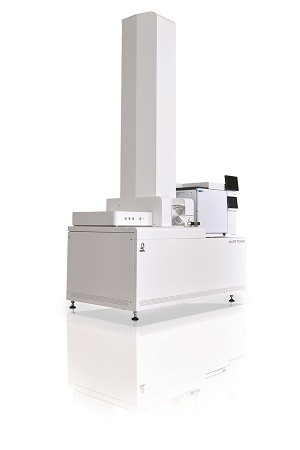Example of a Gas Chromatograph Mass Spectrometer

Now, let’s explain GC/MS using a case study of PAH (Poly Aromatic Hydrocarbon, substances known to be carcinogenic) detected in a lake in North Europe, a region regarded as having the least environmental contamination.

First of all, the organic compound components are extracted from a volume of lake water collected at the site equivalent to about one bottle of beer. The one beer-bottle’s amount of water is concentrated into a quantity for one injection ampule (5ml). The apparently clean water turns into a thick, blackish brown solution after extraction and concentration. It is obvious that it contains many organic compounds. 1μl (1/1,000,000 liter) of this solution is injected into the GC.

A GC is a device to separate each component of the extracted organic compounds (mixture) and to transfer them to the MS. The separation is performed using a capillary column (a slender tube of about 0.3mm inner diameter coated with a solution layer to separate the components. The type of solution layer must be selected according to the purpose). Helium gas is used to carry the sample mixture through the capillary column.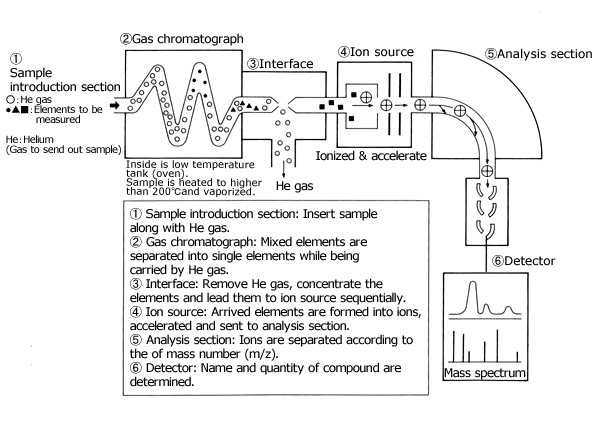#### Details on the picture above

1. Sample Introduction Section: Insert a sample along with He gas.
2. Gas Chromatograph: Mixed components are separated into single components while being transferred by He gas.
3. Interface: Removes He gas, concentrates and introduces the components into the ion source in sequence.
4. Ion Source: The components received are ionized and accelerated, then sent to the analysis section.
5. Analysis Section: Ions are separated by mass number (m/z).
6. Detection Section: The name and quantity of each compound is investigated.
The separation here is quite precise, and even if there are several hundred different components, they will be separated without a mistake. Each component separated by a GC will be transferred to the ion source of the MS separately in sequence, with the helium gases removed by the interface on the way.

The ion source ionizes the received components (state where a molecule is charged with electricity. Here, it means that the electrons typically circling around a molecule are ejected by being struck with an electron coming from outside. This makes the molecule a positive ion, carrying a positive charge). The ionized molecules are accelerated and sent to the analysis section (electric field and magnetic field). There are a variety of ionization methods, including the EI method (electron impact method) using bombardment by electrons, the CI method (chemical ionization method) using chemical reactions, the FD method (field desorption method) using an electric field to induce ionization, a method that is a forte of our company, and the FAB method (fast atom bombardment method) using bombardment by high speed electrons to ionize each component.

Now, a voltage of several kV is applied to each ion of the ionized organic compound, and each ion is accelerated and sent to the analysis section. At the analysis section, each ion is separated by its mass, according to the intensity of the magnetic field as mentioned earlier;, then, they arrive at the detector section. The accuracy of the detection (resolution) is quite precise. To illustrate, instead of saying someone weighs 56kg we are able to say that the weight is 56.9985kg, an accuracy of up to 4 decimal places. In addition, not only weight, but also ”finger prints and wrinkles” (mass spectrum) can be read in 0.1 second. Moreover, the detection sensitivity (minimum quantity that can be reliably detected) is as little as fg order (fg:10-15g)/

This is a much higher sensitivity than is needed to find a single person from among the total population on the globe.

The data detected this way is compared with the data which is already in the computer (library) and printed out as measurement result instantly. As such, each of the components of PAH in the water from the North European Lake has been completely identified, including benzopyrene, a cancer-causing agent.

## Summary of the characteristics of different mass analyzers

All mass spectrometers combine ion formation, mass analysis, and ion detection. This discussion is concerned with how various mass analyzers are used to separate ions according to their mass-to-charge ratio. Each mass analyzer has its own special characteristics and applications and its own benefits and limitations. The choice of mass analyzer should be based upon the application, cost, and performance desired. There is no ideal mass analyzer that is good for all applications. For an excellent and more complete discussion of mass analyzers, see “The Ideal Mass Analyzer: Fact or Fiction?” (Curt Brunnee, Int. J. Mass Spectrom. Ion Proc. 76 (1987), 125-237.

Note that different mass analyzers use different resolution definitions. See “How Resolution is Defined” for a discussion of this topic.

Note also that a complete discussion of MS/MS methods is beyond the scope of this essay, but some comments about MS/MS are provided here in the context of comparing different mass analyzers strengths and weaknesses. See “Tandem Mass Spectrometry MS/MS” for a discussion of this topic.

### General: The effect of electromagnetic fields on ions

All commonly used mass analyzers use electric and magnetic fields to apply a force on charged particles (ions). The relationship between force, mass, and the applied fields can be summarized in Newton’s second law and the Lorentz force law:

F = ma (Newton’s second law)
F = e(E+ v x B) (Lorentz force law)

Where:

• F is the force applied to the ion
• m is the mass of the ion
• a is the acceleration.
• e is the ionic charge
• E is the electric field
• v x B is the vector cross product of the ion velocity and the applied magnetic field

From Newton’s second law, it is apparent that the force causes an acceleration that is mass dependent, and the Lorentz force law tells us that the applied force is also dependent on the ionic charge. Therefore, it should be understood that mass spectrometers separate ions according to their mass-to-charge ratio (m/z) rather than by their mass alone.

### General: The effect of electromagnetic fields on ions

A scanning mass analyzer is analogous to the equipment used in optical spectroscopy for analyzing the color content of visible light. In optical spectroscopy, one starts with visible light which is composed of individual colors (different wavelengths of light) that are present at different intensities. A prism separates the light into its different wavelengths, and a slit is used to select which wavelength reaches the detector. The different wavelengths are then swept (scanned) across the detector slit and the light intensity is recorded as a function of time (wavelength).

In scanning mass spectrometry, one starts with a mixture of ions having different mass-to-charge ratios and different relative abundances. Electromagnetic fields separate the ions according to their mass-to-charge ratios, and a slit is used to select which mass-to-charge ratio reaches the detector. The different mass-to-charge ratios are then scanned across the detector slit and the ion current is recorded as a function of time (mass).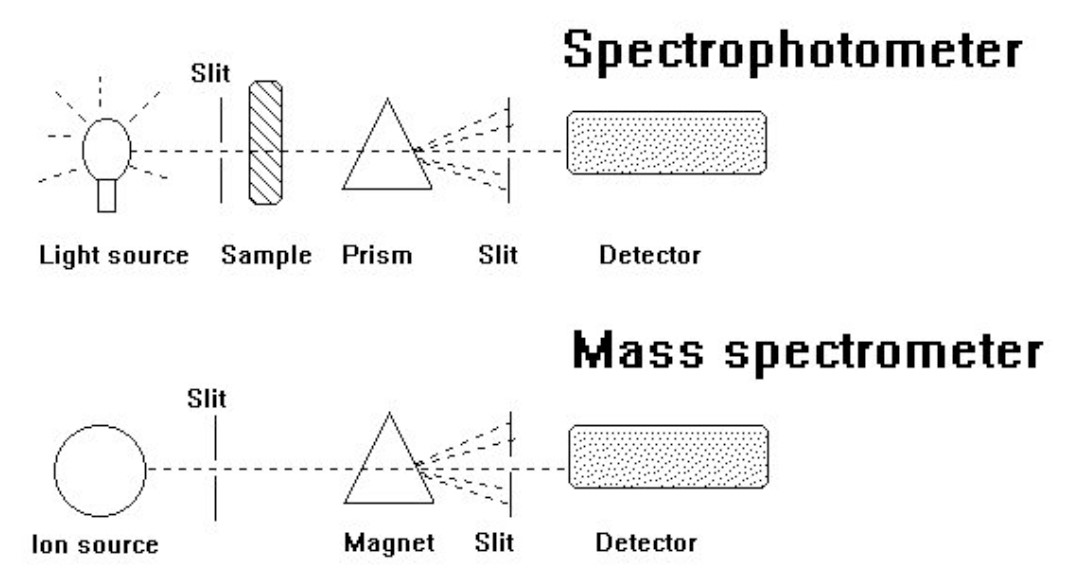Both magnetic sector and quadrupole mass spectrometers can be “jumped” from one target mass to another instead of scanning over a given mass range. This selected ion monitoring (SIM) method is used to improve sensitivity for quantitative analysis ad target compound identification by monitoring only the masses of interest for specific compounds. In contrast to scanning analyses, no time is wasted making measurements of the baseline noise between peaks or measuring ions that are not relevant to the analysis. However, this is only useful when the target masses are known in advance.

## Magnetic Sector Mass Spectrometers

### Principal of operation

The analogy between scanning mass spectrometry and scanning optical spectroscopy is most apparent for magnetic sector mass spectrometers.

In a magnetic deflection mass spectrometer, ions leaving the ion source are accelerated to a high velocity. The ions then pass through a magnetic sector in which the magnetic field is applied in a direction perpendicular to the direction of ion motion. From physics, we know that when acceleration is applied perpendicular to the direction of motion of an object, the object’s velocity remains constant, but the object travels in a circular path. Therefore, the magnetic sector follows an arc; the radius and angle of the arc vary with different ion optical designs.

A magnetic sector alone will separate ions according to their mass-to-charge ratio. However, the resolution will be limited by the fact that ions leaving the ion source do not all have exactly the same energy and therefore do not have exactly the same velocity. This is analogous to the chromatic aberration in optical spectroscopy. To achieve better resolution, it is necessary to add an electric sector that focuses ions according to their kinetic energy. Like the magnetic sector, the electric sector applies a force perpendicular to the direction of ion motion, and therefore has the form of an arc.

A schematic representation of a JEOL double-focusing mass spectrometer is shown below. For historical reasons, this is referred to as a “reverse-geometry” magnetic sector mass spectrometer, which means that the magnetic sector precedes the electric sector.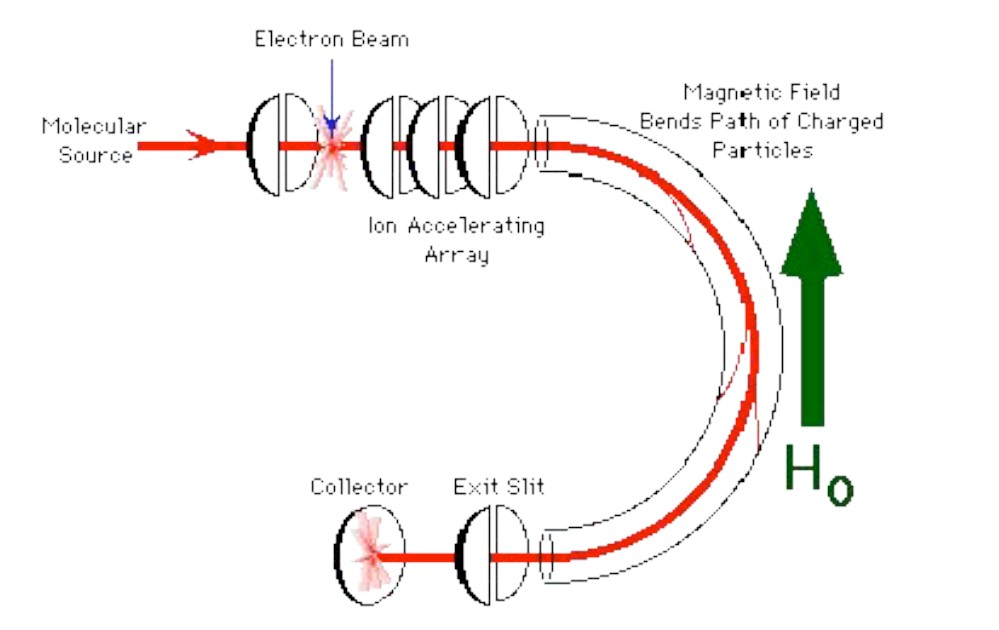The simplest mode of operation of a magnetic sector mass spectrometer keeps the accelerating potential and the electric sector at a constant potential and varies the magnetic field. Ions that have a constant kinetic energy, but different mass-to-charge ratio are brought into focus at the detector slit (called the ‘collector slit”) at different magnetic field strengths.

The dependence of mass-to-charge ratio on the electric and magnetic fields is easily derived. All ion formed in the ion source are accelerated to a kinetic energy, T of: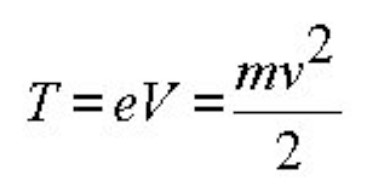Solving for the velocity, v, we get: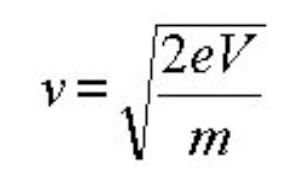From the Lorentz force law, the magnetic field applies a force evB that must be equal to the centripetal force mv**2/r as the ions move in an arc through the magnetic sector: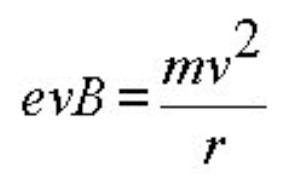Substituting for v, we arrive at the working equation for a magnetic sector mass spectrometer: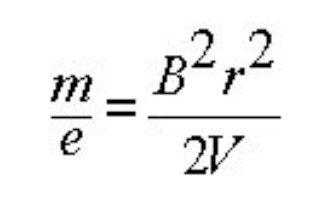As mentioned previously, the electric sector is usually held constant at a value which passes only ions having the specific kinetic energy., V. Therefore, the parameter that is most commonly varied is B, the magnetic field strength. The magnetic field is usually scanned exponentially or linearly to obtain the mass spectrum. A magnetic field scan can be used to cover a wide range of mass-to charge ratios with a sensitivity that is essentially independent of the mass-to-charge ratio.

An alternative is to hold B constant and scan V. The electric sector potential tracks the accelerating voltage. This has the advantage that the electric field is not subject to hysteresis, so the relationship between mass-to-charge ratio and accelerating voltage is a simple linear relationship. The disadvantage of an accelerating voltage (electric field) scan is that the sensitivity is roughly proportional to the mass-to-charge ratio.

The maximum ion transmission and sensitivity occur at the maximum working accelerating voltage for a given magnetic sector mass spectrometer. The effective mass range of the mass spectrometer can be increased by decreasing the accelerating voltage, with a sensitivity that is roughly proportional to the accelerating voltage.

Focal-plane (array) detectors can detect a range of masses simultaneously. This provides a multichannel advantage that can improve the sensitivity for magnetic sectors, and detection limits can be improved if the analysis is limited by the analyte ion current instead of the chemical background level. This is the case for experiments such as MS/MS, electrospray ionization, and field desorption. Array detectors can be used with pulsed ionization methods, but the array detectors for commercial magnetic sector mass spectrometers can only detect a portion of the entire mass range at any given instant.

The resolving power of a magnetic sector mass spectrometer is determined by the slit widths. Higher resolution is obtained by decreasing the slit widths, and thereby decreasing the number of ions that reach the detector.

### Benefits

Double focusing magnetic sector mass analyzers are the “classical” model against which other
mass analyzers are compared.

• Classical mass spectra
• Very high reproducibility
• Best quantitative performance of all mass spectrometer analyzers
• High resolution
• High sensitivity
• High dynamic range
• Linked scan MS/MS does not require another analyzer.
• High-energy CID MS/MS spectra are very reproducible.

### Limitations

• Not well-suited for pulsed ionization methods (e.g., MALDI)
• Usually larger and higher cost than other mass analyzers
• Linked scan MS/MS gives either limited precursor selectivity with unit product-ion resolution, or unit precursor selection with poor product-ion resolution.

### Applications

• All organic MS analysis methods
• Accurate mass measurements
• Quantitation
• Four
• Isotope ratio measurements

### Principal of Operation

The quadrupole mass analyzer is a “mass filter”. Combined DC and RF potentials on the quadrupole rods can be set to pass only a selected mass-to-charge ratio. All other ions do not have a stable trajectory through the quadrupole mass analyzer and will collide with the quadrupole rods., never reaching the detector.

A crude schematic of a quadrupole mass filter is shown below: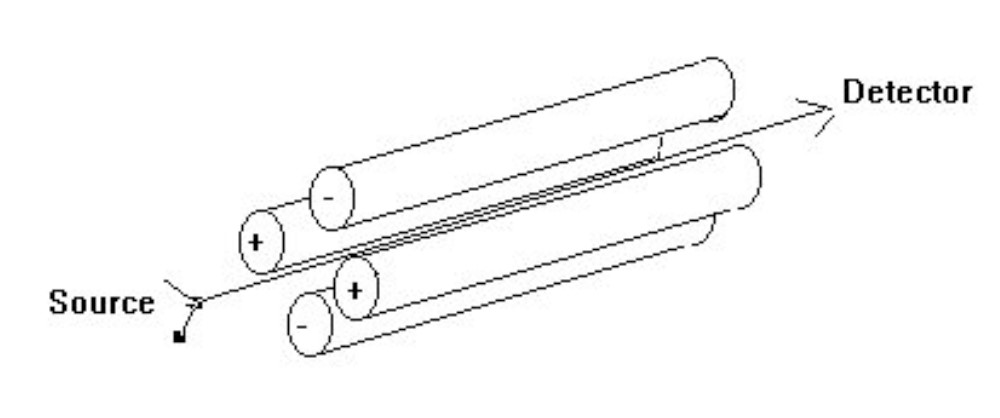A derivation of the working equations for a quadrupole mass analyzer is beyond the scope of this discussion, but it is based upon a second-order differential equation known as the Mathieu equation. See R. E. March, R. J. Hughes and J. F. Todd, “Quadrupole Storage Mass Spectrometry”, volume 102 of Chemical Analysis, Wiley, 1989 for a good description of the theory behind both quadrupole mass filters and quadrupole ion traps.

The operation of a quadrupole mass analyzer is usually treated in terms of a stability diagram that relates the applied DC potential (U) and the applied RF potential (V(t)) and the RF frequency (omega) to a stable vs. unstable ion trajectory through the quadrupole rods. A qualitative representation of a stability diagram for a given mass m is shown below: a and q are parameters that are proportional to U/m and V/m respectively: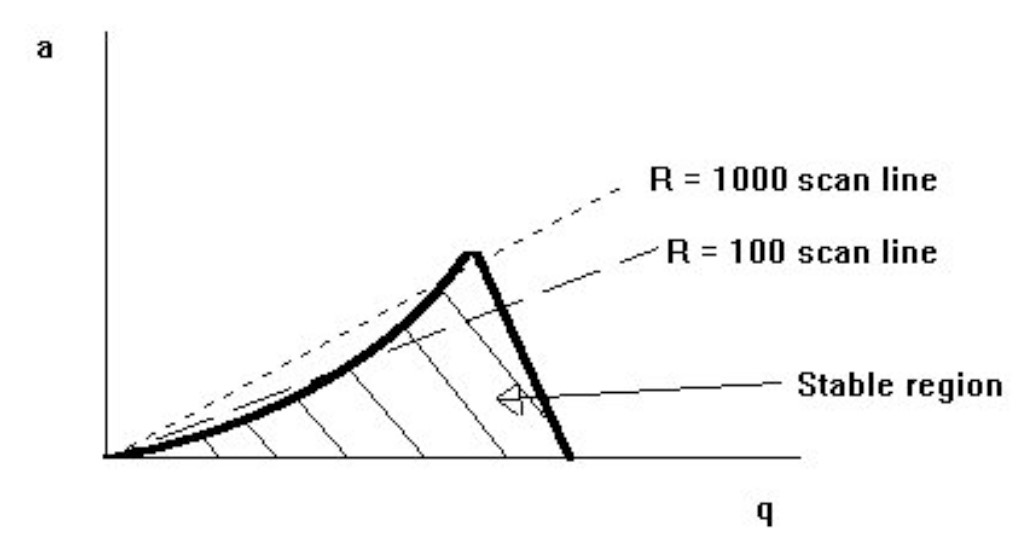Changing the slope of the scan line will change the resolution. Increasing the resolution decreases the number of ions that reach the detector (the region at the apex of the stable region that is bounded by the scan line). Good resolution also depends on the quality of the machining for the quadrupole rods.

Quadrupole rods can have other functions besides their use as a mass filter. An RF-only quadrupole will act as an ion guide for ions within a broad mass range. For example, the collision region of a triple quadrupole mass spectrometer uses an RF ion guide. A DC-only quadrupole is used as a lens element in some ion optic designs (such as JEOL’s magnetic sector mass spectrometers).

### Benefits

• Classical mass spectra
• Good reproducibility
• Relatively small and low-cost systems
• Low-energy collision-induced dissociation (CID) MS/MS spectra in triple quadrupole and hybrid mass spectrometers have efficient conversion of precursor to product.

### Limitations

• Limited resolution
• Peak heights variable as a function of mass (mass discrimination). Peak height vs. mass response must be ‘tuned’.
• Not well suited for pulsed ionization methods
• Low-energy collision-induced dissociation (CID) MS/MS spectra in triple quadrupole and hybrid mass spectrometers depend strongly on energy, collision gas, pressure, and other factors.

### Applications

• Majority of benchtop GC/MS and LC/MS systems
• Sector / quadrupole hybrid MS/MS systems

## Time-of-Flight Mass Analyzers

### Principal of Operation

#### Linear

A time-of-flight mass spectrometer measures the mass-dependent time it takes ions of different masses to move from the ion source to the detector. This requires that the starting time (the time at which the ions leave the ion source) is well-defined. Therefore, ions are either formed by a pulsed ionization method (usually matrix-assisted laser desorption ionization, or MALDI), or various kinds of rapid electric field switching are used as a ‘gate’ to release the ions from the ion source in a very short time.

Recall that the kinetic energy of an ion leaving the ion source is:The ion velocity, v, is the length of the flight path, L , divided by the flight time, t: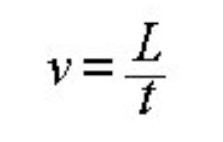Substituting this expression for v into the kinetic energy relation, we can derive the working equation for the time-of-flight mass spectrometer: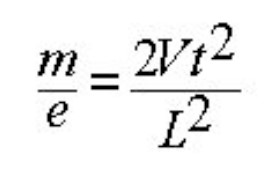or, rearranging the equation to solve for the time-of-flight: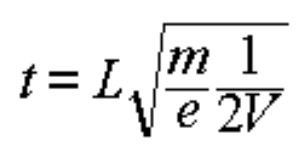### Reflectron

The ions leaving the ion source of a time-of-flight mass spectrometer have neither exactly the same starting times nor exactly the same kinetic energies (recall the “chromatic aberrations” discussed for magnetic sector mass spectrometers). Various time-of-flight mass spectrometer designs have been developed to compensate for these differences. A reflectron is an ion optic device in which ions in a time-of-flight mass spectrometer pass through a “mirror” or “reflectron” and their flight is reversed.

A linear field reflectron allows ions with greater kinetic energies to penetrate deeper into the reflectron than ions with smaller kinetic energies. The ions that penetrate deeper will take longer to return to the detector. If a packet of ions of a given mass-to-charge ratio contains ions with varying kinetic energies, then the reflectron will decrease the spread in the ion flight times, and therefore improve the resolution of the time-of-flight mass spectrometer.

A curved-field reflectron ensures that the ideal detector position for the time-of-flight mass spectrometer does not vary with mass-to-charge ratio. This also results in improved resolution for time-of-flight mass spectrometers.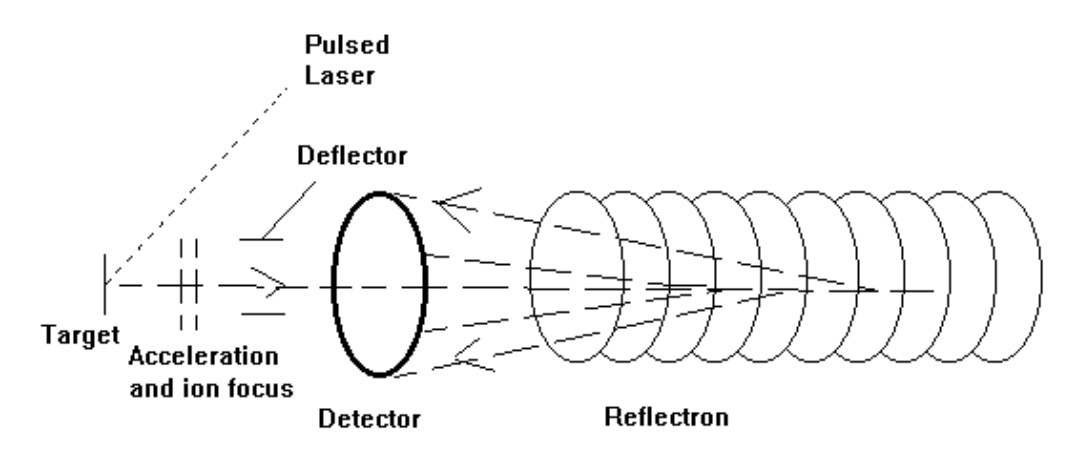### Benefits

• Fastest MS analyzer
• Well suited for pulsed ionization methods (method of choice for majority of MALDI mass spectrometer systems)
• High ion transmission
• MS/MS information from post-source decay
• Highest practical mass range of all MS analyzers

### Limitations

• Requires pulsed ionization method or ion beam switching (duty cycle is a factor)
• Fast digitizers used in TOF can have limited dynamic range
• Limited precursor-ion selectivity for most MS/MS experiments

### Applications

• Almost all MALDI systems
• Very fast GC/MS systems

## Trapped-Ion Mass Analyzers

There are two principal trapped-ion mass analyzers: three-dimensional quadrupole ion traps (“dynamic” traps), and ion cyclotron resonance mass spectrometers (“static” traps). Both operate by storing ions in the trap and manipulating the ions by using DC and RF electric fields in a series of carefully timed events. This provides some unique capabilities, such as extended MS/MS experiments, very high resolution, and high sensitivity. The tradeoff is that trapping the ions for long periods of time (milliseconds to days) provides plenty of time for the ions fall apart spontaneously (unimolecular decomposition), to experience undesirable interactions with other ions (space charge effects), neutral molecules (ion-molecule reactions), or perturbations in the ion motion due to imperfect electric fields. This can lead to artifacts and unexpected changes in the mass spectrum (so called “non-classical mass spectra”).

### Ion Cyclotron Resonance

#### Principal of Operation

Ions move in a circular path in a magnetic field. The cyclotron frequency of the ion’s circular motion is mass dependent. By measuring the cyclotron frequency, one can determine an ion’s mass. The working equation for ICR can be quickly derived by equating the centripetal force (mv**2/r) and the Lorentz force evB experienced by an ion in a magnetic field: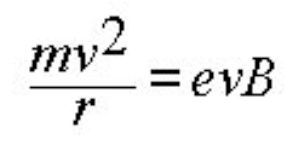Solving for the angular frequency (omega), which is equal to v/r :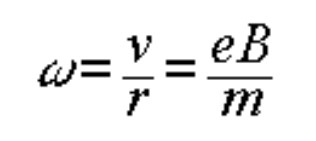A group of ions of the same mass-to-charge ratio will have the same cyclotron frequency, but they will be moving independently and out-of-phase at roughly thermal energies. If an excitation pulse is applied at the cyclotron frequency, the “resonant” ions will absorb energy and be brought into phase with the excitation pulse. As ions absorb energy, the size of their orbit also increases. The packet of ions passes close to the receiver plates in the ICR cell and induces image currents that can be amplified and digitized. The signal induced in the receiver plates depends on the number of ions and their distance from the receiver plates.

If several different masses are present, then one must apply an excitation pulse that contains components at all of the cyclotron frequencies. This is done by using a rapid frequency sweep (“chirp”), an “impulse” excitation, or a tailored waveform. The image currents induced in the receiver plates will contain frequency components from all of the mass-to-charge ratios. The various frequencies and their relative abundances can be extracted mathematically by using a Fourier transform which converts a time-domain signal (the image currents) to a frequency-domain spectrum (the mass spectrum).

A schematic representation of a cubic FTICR cell is shown below: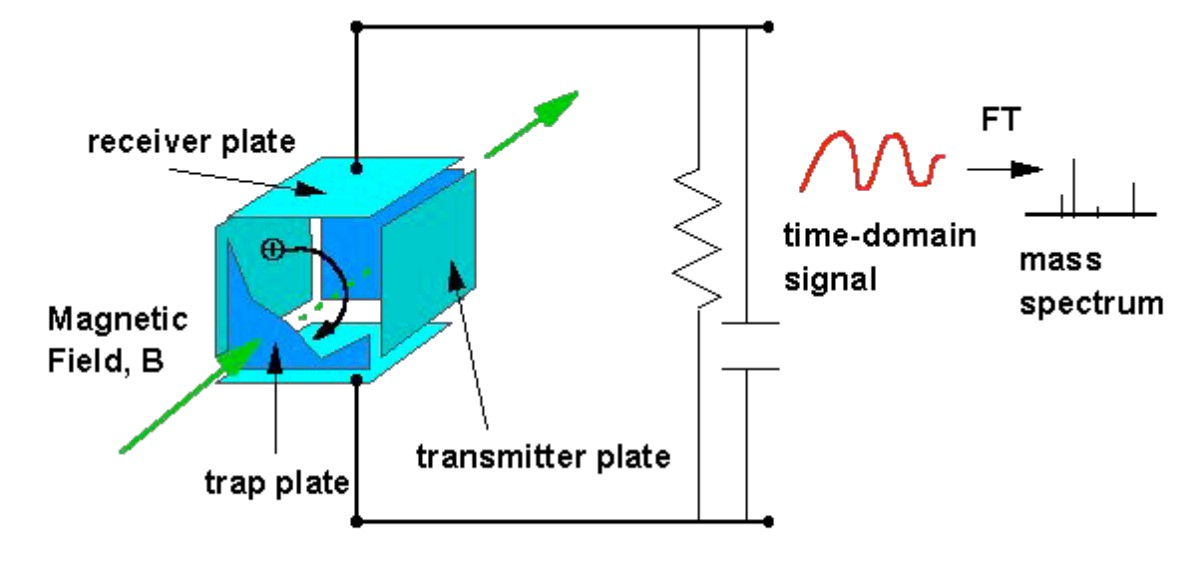The cubic ICR cell consists of three pairs of parallel plates. The functions of the excitation and receiver plates are apparent from the preceding discussion. A small potential is applied to the trapping plates to keep the ions contained within the ICR cell because the magnetic field does not constrain the ion motion along the direction of the applied magnetic field. Beside the cubic cell, many other ICR cell designs have been evaluated, and each has its own special characteristics.

Excitation events can be used to increase the kinetic energy of ions, or to eject ions of a given mass-to-charge ratio from the cell by increasing the orbital radius until ions are lost by collisions with the cell plates.

The background pressure of an FTICR should be very low to minimize ion-molecule reactions and ion-neutral collisions that damp the coherent ion motion. A variety of external ion source designs have been developed to deal with this problem, and each design has its own performance characteristics.

Most FTICR mass spectrometers use superconducting magnets, which provide a relatively stable calibration over a long period of time. Although some mass accuracy can be obtained without internal calibrant, mass accuracy and resolution are inversely proportional to m/z, and the best accuracte mass measurements require an internal calibrant.

Unlike the quadrupole ion trap, the FTICR mass spectrometer is not operated as a scanning device.

### Benefits

• The highest recorded mass resolution of all mass spectrometers
• Powerful capabilities for ion chemistry and MS/MS experiments
• Well-suited for use with pulsed ionization methods such as MALDI
• Non-destructive ion detection; ion remeasurement
• Stable mass calibration in superconducting magnet FTICR systems

### Limitations

• Limited dynamic range
• Strict low-pressure requirements mandate an external source for most analytical applications
• Subject to space charge effects and ion molecule reactions
• Artifacts such as harmonics and sidebands are present in the mass spectra
• Many parameters (excitation, trapping, detection conditions) comprise the experiment sequence that defines the quality of the mass spectrum
• Generally low-energy CID, spectrum depends on collision energy, collision gas, and other parameters

### Applications

• Ion chemistry
• High-resolution MALDI and electrospray experiments for high-mass analytes
• Laser desorption for materials and surface characterization

### Principal of Operation

Ions are dynamically stored in a three-dimensional quadrupole ion storage device. The RF and DC potentials can be scanned to eject successive mass-to-charge ratios from the trap into the detector (mass-selective ejection). The theory of the ion trap is beyond the scope of this essay, and the reader is again referred to R. E. March, R. J. Hughes and J. F. Todd, “Quadrupole Storage Mass Spectrometry”, volume 102 of Chemical Analysis, Wiley, 1989 for a good description of the theory behind both quadrupole mass filters and quadrupole ion traps.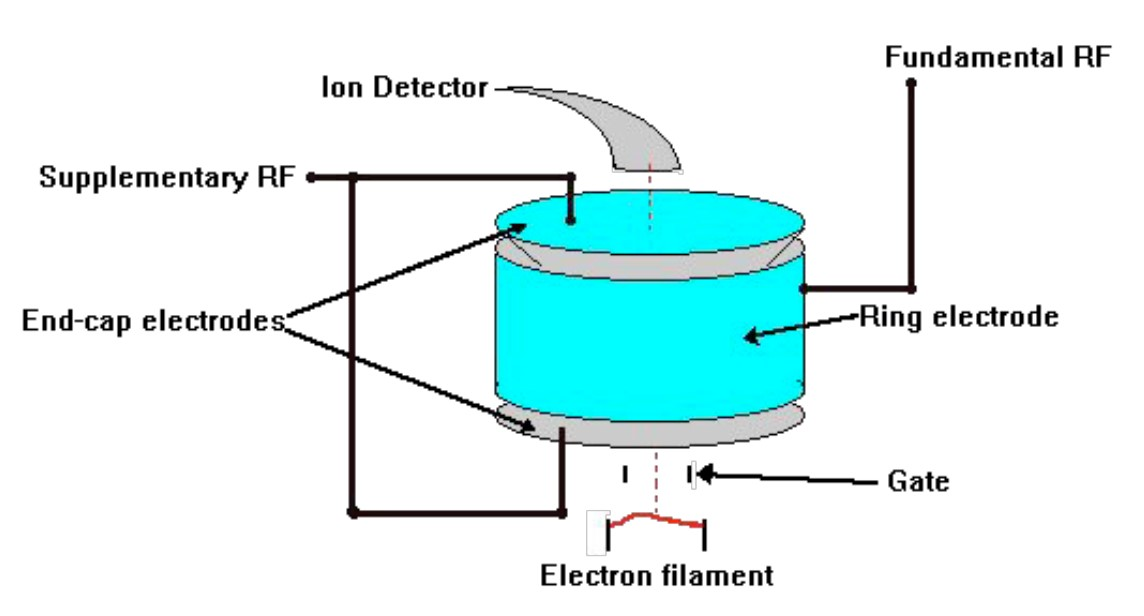Ions are formed within the ion trap or injected into an ion trap from an external source. The ions are dynamically trapped by the applied RF potentials (a common trap design also makes use of a “bath gas” to help contain the ions in the trap). The trapped ions can be manipulated by RF events analogous to the events in FTICR to perform ion ejection, ion excitation, and massselective ejection. This provides MS/MS and MS/MS/MS… experiments analogous to those performed in FTICR.

Space-charge effects (ion-ion repulsion) severely limit the inherent dynamic range of the ion trap. This is usually handled by auto-ranging. That is, a pre-scan is performed to determine the ion current, and then the ionizing electron current is adjusted to reduce the number of ions formed to within the working range. This can be done wherever the ion formation event can be manipulated to control the number of ions formed (such as in electron ionization).

The ions contained within the ion trap can react with any neutral species present. “Self-CI” refers to the case when analyte ions react with analyte neutrals within the trap, and this can produce concentration-dependent changes in the mass spectrum. Such effects can be reduced by injecting ions into the trap from an external source, but ion transmission and trapping losses can lead to reduced sensitivity compared to ion traps that form ions within the trap.

Researchers have demonstrated high resolution and high mass range in specially designed ion trap systems. However, these extended capabilities have not been made generally available in commercial ion trap systems.

### Benefits

• High sensitivity
• Multi-stage mass spectrometry (analogous to FTICR experiments)
• Compact mass analyzer

### Limitations

• Poor quantitation
• Very poor dynamic range (can sometimes be compensated for by using auto-ranging)
• Subject to space charge effects and ion molecule reactions
• Collision energy not well-defined in CID MS/MS
• Many parameters (excitation, trapping, detection conditions) comprise the experiment sequence that defines the quality of the mass spectrum.

### Applications

• Benchtop GC/MS, LC/MS and MS/MS systems
• Target compound screening
• Ion chemistry
JEOL Mass Spectrometer Solutions for Today’s Analytical Challenges
Our complete line of mass spectrometer systems along with our years of experience can help you solve problems in a wide variety of areas, including environmental, forensics, petroleum, chemicals, and polymer analysis.